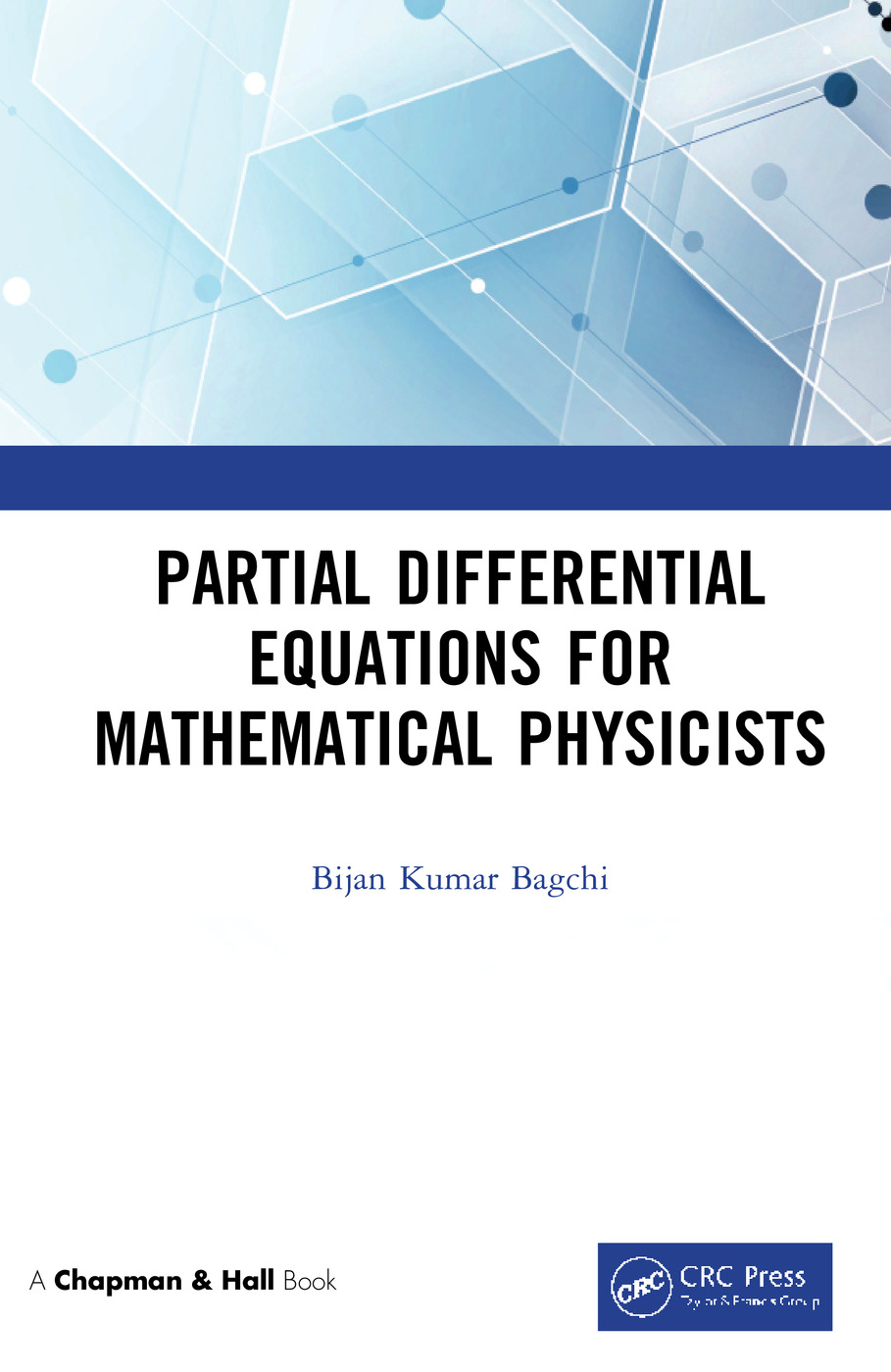# Partial Differential Equations for Mathematical Physicists

## 1st Edition

Chapman and Hall/CRC

224 pages | 20 B/W Illus.

##### Purchasing Options:\$ = USD
Hardback: 9780367227029
pub: 2019-07-16
SAVE ~\$23.99
Available for pre-order
\$119.95
\$95.96
x

FREE Standard Shipping!

### Description

This book is intended for graduate students, researchers of theoretical physics and applied mathematics, and professionals who want to undertake a course-work in partial di erential equations. It gives all the essentials of the subject with the only prerequisites are an elementary knowledge of introductory calculus, ordinary di erential equations and certain aspects of classical mechanics. We have laid greater stress on the methodologies of partial di erential equations and how they can be implemented as tools for extracting their solutions rather than trying to dwell on the foundational aspects. After covering some basic materials the book proceeds to focus mostly on the three main types of second order linear equations, namely those belonging to the elliptic, hyperbolic and parabolic class. For such equations a detailed treatment is given of the derivation of Green's functions, of the role of characteristics and of techniques required in handling the solutions with the expected amount of rigor. In this regard we have discussed at length the method of separation variables, application of Green's function technique and employment of Fourier and Laplace's transforms. Also collected in the appendices are some useful results from the Dirac delta function, Fourier transform and Laplace transform meant to be used

as supplementary materials to the text. A good number of problems are worked out and equally a large number of exercises have been appended at the end of each chapter keeping in mind the needs of the students. It is expected that the book would provide a systematic and unitary coverage of the basics of partial di erential equations.

Key Features

• An adequate and substantive exposition of the subject.
• Covers a wide range of important topics.
• Maintains throughout mathematical rigor.
• Organizes materials in a self-contained way with each chapter ending with a summary.
• Contains a large number of worked out problems.

### Table of Contents

Preface. Author Bio. Preliminary concepts and background material. Basic properties of a second order linear PDE. PDE: The elliptic form. PDE: The hyperbolic form. PDE: The parabolic form. Solving PDEs by the integral transform method. A Dirac delta function. B Fourier transform. C Laplace transform. Bibliography. Index.

### About the Author

Bijan Bagchi received his B.Sc., M.Sc., and Ph.D. degrees from the University of Calcutta. He has a variety of research interests and involvements ranging from spectral problems in quantum mechanics to exactly solvable models, supersymmetric quantum mechanics, parity-time- symmetry and related non-Hermitian phenomenology, nonlinear dynamics, integrable models and high energy phenomenology. He has published more than 150 research articles in refereed journals and held a number of international visiting positions. He is the author of the books entitled Advanced Classical Mechanics and Supersymmetry in Quantum and Classical Mechanics both published by CRC respectively in the years 2017 and 2000. He was formerly a Professor in Applied Mathematics at the University of Calcutta and currently a Professor in the Department of Physics at Shiv Nadar University.

### Subject Categories

##### BISAC Subject Codes/Headings:
MAT000000
MATHEMATICS / General
MAT004000
MATHEMATICS / Arithmetic
MAT007000
MATHEMATICS / Differential Equations
SCI040000
SCIENCE / Mathematical Physics